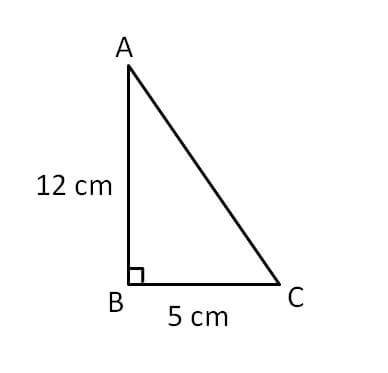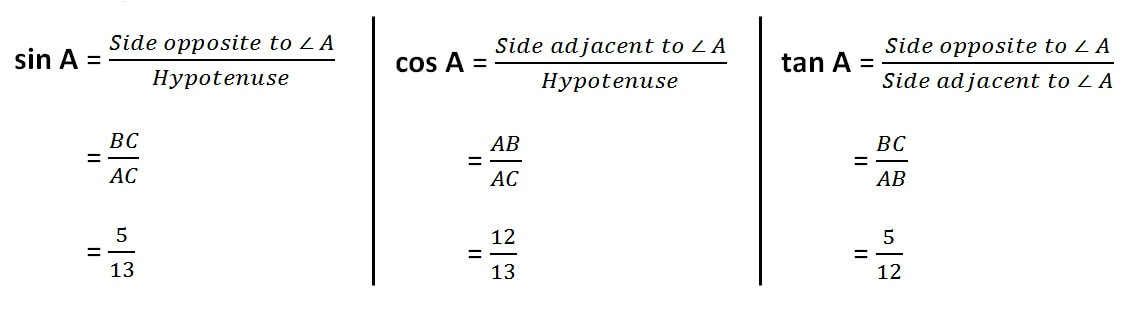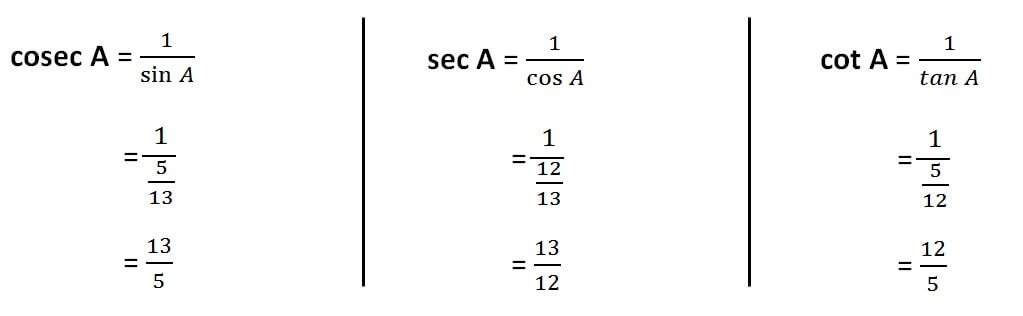Finding sin cos when sides of a triangle are given

Chapter 8 Class 10 Introduction to Trignometry
Concept wise#### In Δ ABC, right angled at B. AB = 12 cm, BC = 5cm.

Find sin A, cos A, tan A, cosec A, sec A, cot A

First we draw the triangle

Step 1 : Finding sides of triangle

In right triangle ABC,

Using Pythagoras theorem

(Hypotenuse) 2  = (Height) 2  + (Base) 2

AC 2   = AB 2  + BC 2

AC 2  = 122 + 52

AC 2  = 144 + 25

AC 2   = 169

AC = √169

AC = √(13 ×13) =√(13 2 ) = 13

Hence AC = 13 cm

So, our triangle becomes

Step 2 : Finding sin A , cos A, tan ANow, let us find cosec, cot and tanLearn in your speed, with individual attention - Teachoo Maths 1-on-1 Class